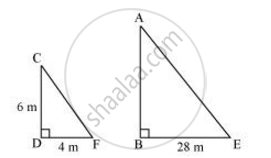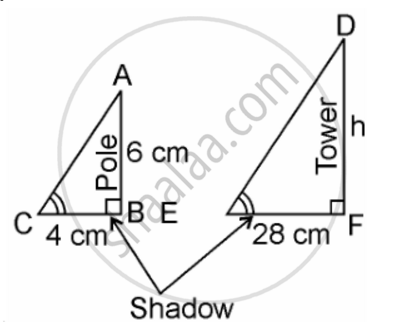# A vertical pole of a length 6 m casts a shadow 4m long on the ground and at the same time a tower casts a shadow 28 m long. Find the height of the tower. - Mathematics

A vertical pole of a length 6 m casts a shadow 4m long on the ground and at the same time a tower casts a shadow 28 m long. Find the height of the tower.

#### Solution 1Let AB and CD be a tower and a pole respectively.

Let the shadow of BE and DF be the shadow of AB and CD respectively.

At the same time, the light rays from the sun will fall on the tower and the pole at the same angle.

Therefore, ∠DCF = ∠BAE

And, ∠DFC = ∠BEA

∠CDF = ∠ABE (Tower and pole are vertical to the ground)

∴ ΔABE ∼ ΔCDF (AAA similarity criterion)

=> (AB)/(CB) = (BE)/(DF)

=> (AB)/(6m) = 28/4

=> AB = 42 m

Therefore, the height of the tower will be 42 metres.

#### Solution 2Length of the vertical pole = 6m (Given)
Shadow of the pole = 4 m (Given)
Let Height of tower = h m
Length of shadow of the tower = 28 m (Given)
In ΔABC and ΔDEF,
∠C = ∠E (angular elevation of sum)
∠B = ∠F = 90°
∴ ΔABC ~ ΔDEF (By AA similarity criterion)
∴ AB/DF = BC/EF (If two triangles are similar corresponding sides are proportional)
∴ 6/h = 4/28
⇒ h = 6×28/4
⇒ h = 6 × 7
⇒ = 42 m
Hence, the height of the tower is 42 m
Concept: Criteria for Similarity of Triangles
Is there an error in this question or solution?

#### APPEARS IN

NCERT Class 10 Maths
Chapter 6 Triangles
Exercise 6.3 | Q 15 | Page 141